ISEE Lower Level Quantitative : How to find the area of a triangle

Example Questions

1 2 3 4 5 7 Next →

Example Question #103 : Triangles

What is the area of the right triangle in the following figure?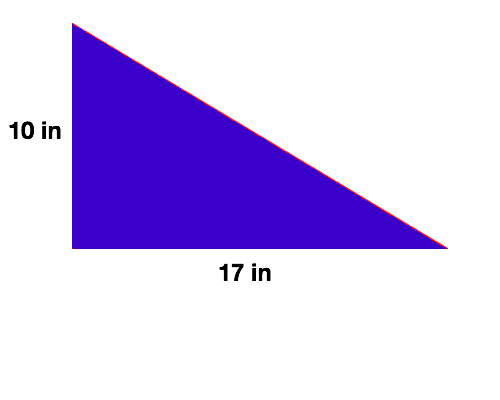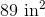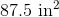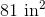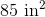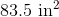Explanation:

There are several different ways to solve for the area of a right triangle. In this lesson, we will transform the right triangle into a rectangle, use the the simpler formula for area of a rectangle to solve for the new figure's area, and divide this area in half in order to solve for the area of the original figure.

First, let's transform the triangle into a rectangle: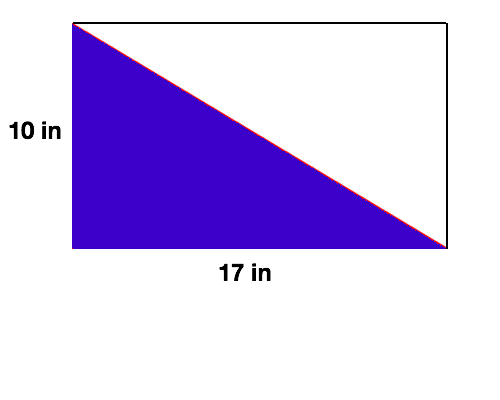Second, let's remember that the formula for area of a rectangle is  as follows: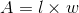Substitute in our side lengths.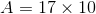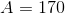Last, notice that our triangle is exactly half the size of the rectangle that we made. This means that in order to solve for the area of the triangle we will need to take half of the area of the rectangle, or divide it by.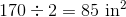Thus, the area formula for a right triangle is as follows: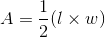or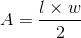Example Question #104 : Triangles

What is the area of the right triangle in the following figure?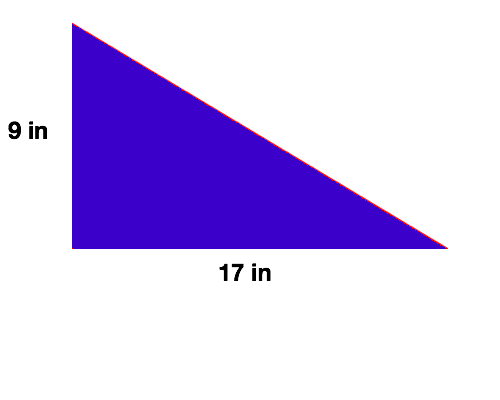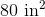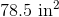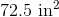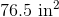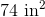Explanation:

There are several different ways to solve for the area of a right triangle. In this lesson, we will transform the right triangle into a rectangle, use the the simpler formula for area of a rectangle to solve for the new figure's area, and divide this area in half in order to solve for the area of the original figure.

First, let's transform the triangle into a rectangle: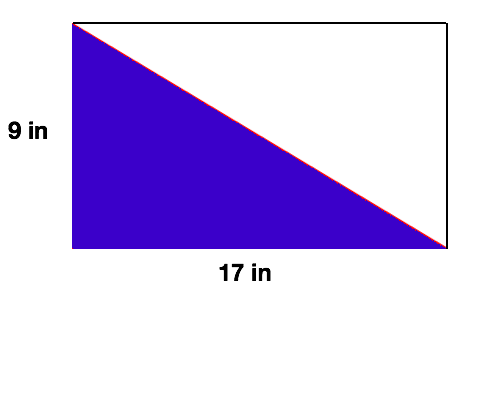Second, let's remember that the formula for area of a rectangle is  as follows:Substitute in our side lengths.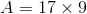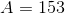Last, notice that our triangle is exactly half the size of the rectangle that we made. This means that in order to solve for the area of the triangle we will need to take half of the area of the rectangle, or divide it by.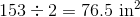Thus, the area formula for a right triangle is as follows:orExample Question #105 : Triangles

What is the area of the right triangle in the following figure?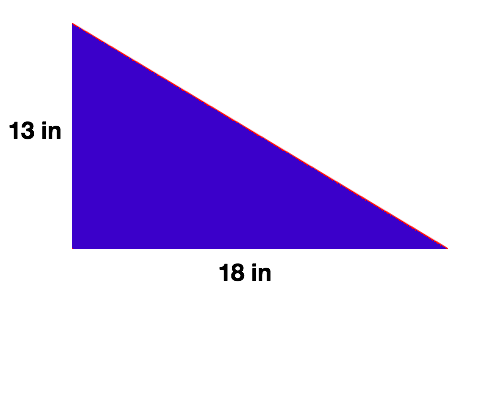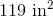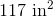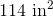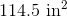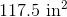Explanation:

There are several different ways to solve for the area of a right triangle. In this lesson, we will transform the right triangle into a rectangle, use the the simpler formula for area of a rectangle to solve for the new figure's area, and divide this area in half in order to solve for the area of the original figure.

First, let's transform the triangle into a rectangle: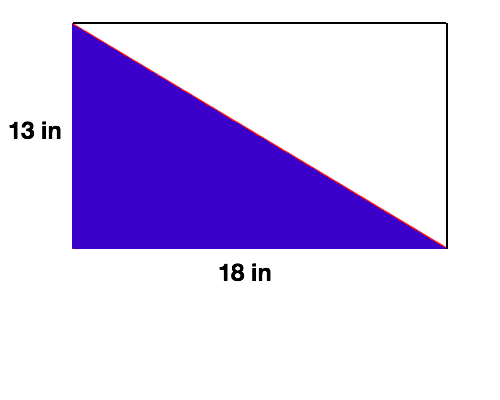Second, let's remember that the formula for area of a rectangle is  as follows:Substitute in our side lengths.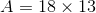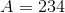Last, notice that our triangle is exactly half the size of the rectangle that we made. This means that in order to solve for the area of the triangle we will need to take half of the area of the rectangle, or divide it by.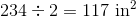Thus, the area formula for a right triangle is as follows:orExample Question #106 : Triangles

What is the area of the right triangle in the following figure?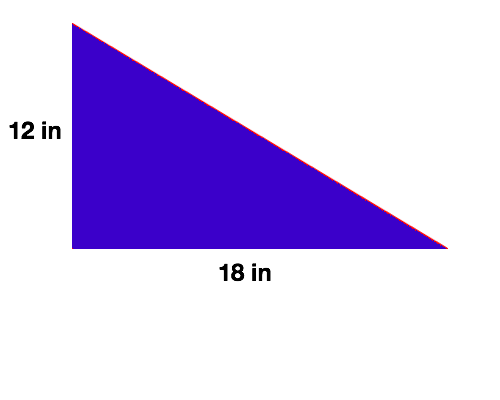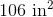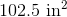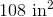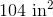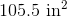Explanation:

There are several different ways to solve for the area of a right triangle. In this lesson, we will transform the right triangle into a rectangle, use the the simpler formula for area of a rectangle to solve for the new figure's area, and divide this area in half in order to solve for the area of the original figure.

First, let's transform the triangle into a rectangle: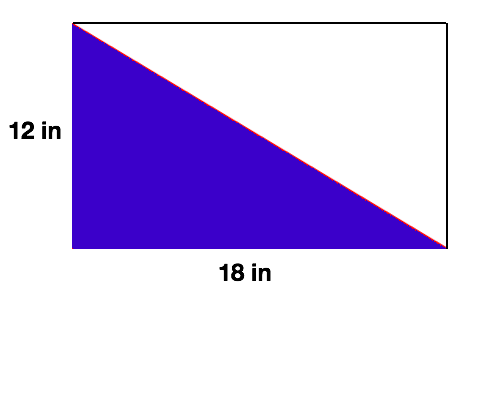Second, let's remember that the formula for area of a rectangle is  as follows:Substitute in our side lengths.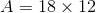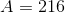Last, notice that our triangle is exactly half the size of the rectangle that we made. This means that in order to solve for the area of the triangle we will need to take half of the area of the rectangle, or divide it by.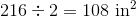Thus, the area formula for a right triangle is as follows:orExample Question #107 : Triangles

What is the area of the right triangle in the following figure?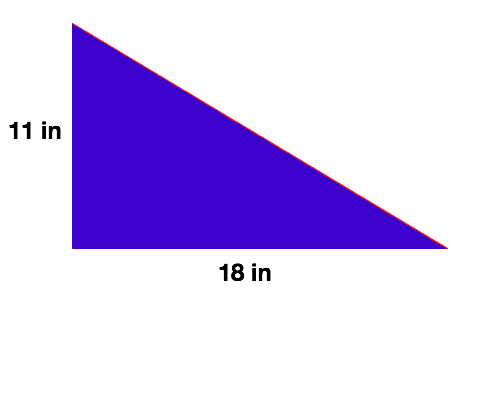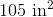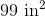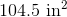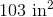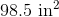Explanation:

There are several different ways to solve for the area of a right triangle. In this lesson, we will transform the right triangle into a rectangle, use the the simpler formula for area of a rectangle to solve for the new figure's area, and divide this area in half in order to solve for the area of the original figure.

First, let's transform the triangle into a rectangle: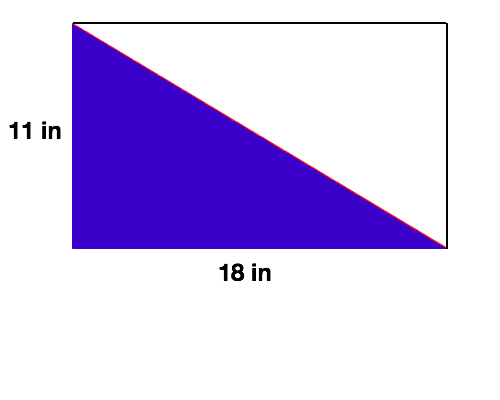Second, let's remember that the formula for area of a rectangle is  as follows:Substitute in our side lengths.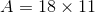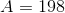Last, notice that our triangle is exactly half the size of the rectangle that we made. This means that in order to solve for the area of the triangle we will need to take half of the area of the rectangle, or divide it by.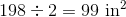Thus, the area formula for a right triangle is as follows:orExample Question #108 : Triangles

What is the area of the right triangle in the following figure?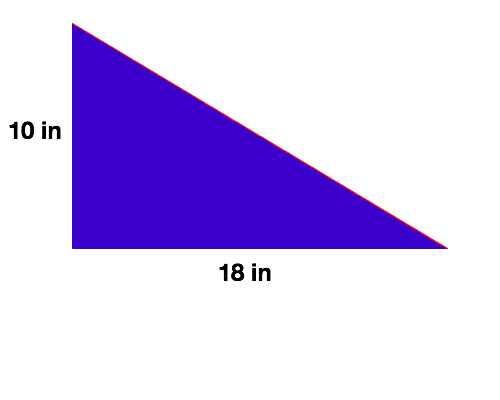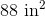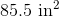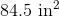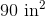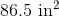Explanation:

There are several different ways to solve for the area of a right triangle. In this lesson, we will transform the right triangle into a rectangle, use the the simpler formula for area of a rectangle to solve for the new figure's area, and divide this area in half in order to solve for the area of the original figure.

First, let's transform the triangle into a rectangle: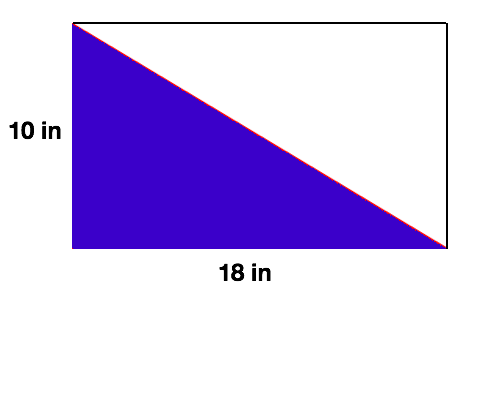Second, let's remember that the formula for area of a rectangle is  as follows:Substitute in our side lengths.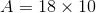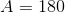Last, notice that our triangle is exactly half the size of the rectangle that we made. This means that in order to solve for the area of the triangle we will need to take half of the area of the rectangle, or divide it by.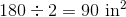Thus, the area formula for a right triangle is as follows:or1 2 3 4 5 7 Next →